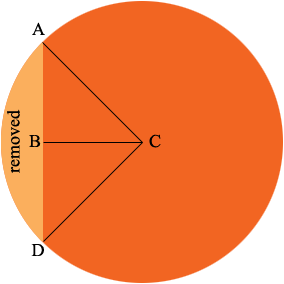SEARCH HOMEMath Central Quandaries & QueriesQuestion from heather, a teacher: The height of the silo is 30ft and the face that rests against a barn is 10 ft wide. If the barn if 5 ft from the center of the silo what is the capacity of the silo?Hi Heather,

The volume of the silo is the area of the base times the height. Here is my diagram of the base.|AB| = |BC| = 5 feet so an application of Pythagoras theorem to the triangle ABC will give you |CA|, the radius of the circle. You can now find the area of the circle and all that remains is to calculate the area of the portion removed.

Since |AB| = |BC| the triangle ABC is an isosceles right triangle and hence the measure of the angle BCA is 45o. Thus angle DCA is a right angle and thus the sector CAD is one quarter of the circle. You can calculate the area of the triangle DCA and thus the area of the removed portion of the circle is one quarter the area of the circle minus the area of the triangle DCA.

PennyMath Central is supported by the University of Regina and The Pacific Institute for the Mathematical Sciences.